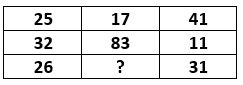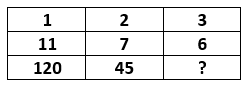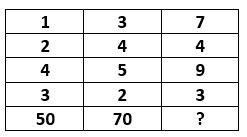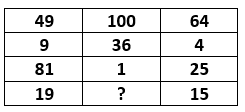# Number Puzzles | Logical Puzzles with Answers - Reasoning MCQs for SSC Exam

Questions

### Find the missing term in each of the following:

1A 13
B 15
C 16
D 19

Solution:

(11 + 25) - 17 = 36 - 17 = 19

(6 + 34) - 12 = 40 - 12 = 28

(8 + 19) - x = 11 ⇒ x = 27 - 11 = 16

2A 22
B 26
C 30
D 34

Solution:

Number at the centre is the sum of the numbers in the row and column.

83 - (25 + 41) = 83 - 66 = 17

83 - (25 + 26) = 83 - 51 = 32

83 - (41 + 31) = 83 - 72 = 11

So, missing number = 83 - (26 + 31) = 83 - 57 = 26

3A 23
B 25
C 27
D 29

Solution:

112 - 12 = 121 - 1 = 120

72 - 22 = 49 - 4 = 45

Hence, missing number = 62 - 32 = 36 - 9 = 27

4A 5
B 8
C 11
D 13

Solution:

Number in third column = 2 × Number in first column + 3 × Number in Second column

(2 × 8) + (17 × 3) = 67

(13 × 2) + (11 × 3) = 59

So, (x × 2) + (13 × 3) = 49 ⇒ x × 2 = 49 - 39 ⇒ x = 5

5A 5
B 10
C 8
D 6

Solution:

5 × 4 × 8 = 160

7 × 8 × 6 = 336

So, missing number = 9 × 2 × x = 108 ⇒ x = 108 ÷ 18 = 6

6A 110
B 115
C 120
D 125

Solution:

(1 + 2 + 4 + 3) × 5 = 50

(3 + 4 + 5 + 2) × 5 = 70

So, missing number (7 + 4 + 9 + 3) × 5 = 115

7A 15
B 16
C 17
D 18

Solution:### Chapters